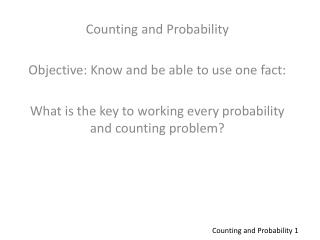DownloadDownload PresentationCounting and Probability Objective: Know and be able to use one fact:

Counting and Probability Objective: Know and be able to use one fact:

Download PresentationCounting and Probability Objective: Know and be able to use one fact:

- - - - - - - - - - - - - - - - - - - - - - - - - - - E N D - - - - - - - - - - - - - - - - - - - - - - - - - - -
Presentation Transcript

1. Counting and Probability Objective: Know and be able to use one fact: What is the key to working every probability and counting problem? Counting and Probability 1

2. Of the 410 students at Kennedy High School, 240 study Spanish and 180 study French. If 25 students study neither language, how many students study both? Counting and Probability 1

3. A is the set of positive integers less than 20; B is the set of positive integers that contain the digit 7; and C is the set of primes. How many numbers are members of the region labeled x. A B C X Y Counting --2

4. In 2003, Thanksgiving was on Thursday, November 27, and there are 30 days in November. If one day in November 2003 was chosen at random for a concert, what is the probability that the concert was on a weekend (Saturday or Sunday)? Counting and Probability 3

5. Tim has a six individual red socks and two individual blue socks and two individual black socks in a draw? What is the probability that Tim randomly reaches into his draw and picks one red sock? Counting and Probability 4

6. Tim has a six individual red socks and two individual blue socks and two individual black socks in a draw? What is the probability that Tim randomly reaches into his draw and picks two red socks? Counting and Probability 5

7. Tim has a six individual red socks and two individual blue socks and two individual black socks in a draw? What is the probability that Tim randomly reaches into his draw and picks one red or one blue sock? Counting and Probability 6

8. An integer between 100 and 999, inclusive, is chosen at random. What is the probability that all the digits of the number are odd? Counting and Probability 7

9. An integer between 100 and 999, inclusive, is chosen at random. What is the probability that all the digits of the number are odd? Counting and Probability 8

10. In the figure below, a white square whose sides are 4 has been pasted on a black square whose sides are 5. If a point is chosen at random from the large square, what is the probability that the point is in the black area? Counting and Probability 9

11. In the figure below, each of the small circles has a radius of 2 and the large circle has a radius of 6. If a point is chosen at random inside the large circle, what is the probability that the point lies in the shaded region? Counting and Probability 10

12. A Special Problem A jar has 5 marbles, 1 of each of the colors red, white, blue, green and yellow. If 4 marbles are removed from the jar, what is the probability that the yellow marble was removed? Counting and Probability 11